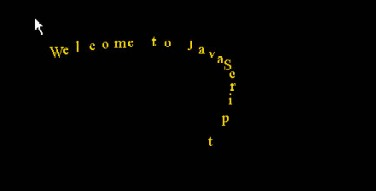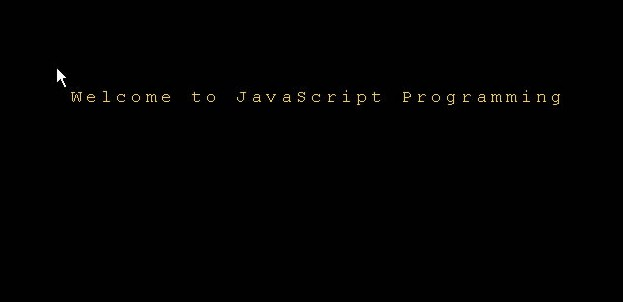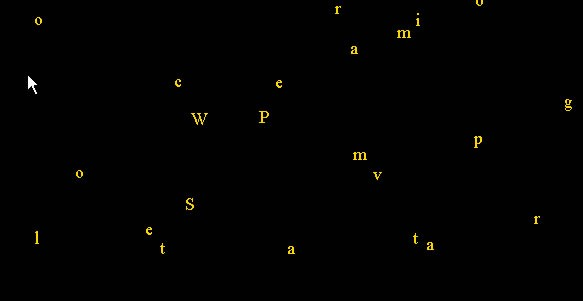## Creating Animated Text for Web Pages Using Difference Equations and JavaScript

Paul Bouthellier
Department of Mathematics and Computer Science
University of Pittsburgh-Titusville
Titusville, PA 16354
FAX: 814-827-5574
Ph: 814-827-4432
E-mail: pbouthe@pitt.edu

Javascript is a programming language that is used on web pages. In various classes I have used Javascript to created business calculators, numerical analysis pages using Newton's method and interpolation polynomials, rotations of images using trigonometry, and dynamic pages using difference equations. In classes ranging from algebra to difference equations I have used slopes, trigonometry, and difference equations to animate text and images on web pages.

Using Javascript to create dynamic effects on web pages allows students to study the effects of changes of the equations used to animate the elements of the page. Even if a student does not have any math packages at home, they generally have a computer on which they can run their web pages and continue their animation studies.

In this paper we shall shall look at several examples in which text is broken into individual letters and placed on separate layers. The coordinates of each layer is controlled by the output of difference equations, one for the x-coordinate and another for the y-coordinate.

For the instructor, in any Javascript project perhaps the most important part is to write a template for the Javascript program that will accomplish the mathematical goals while being as simple as possible. The students should be able to concentrate on the math-not on the syntax of the language. This will often take a good deal of time and a number of iterations.

Difference Equations-Stability and InstabilityThe following is part of the Javascript program needed to animate the text "Welcome to JavaScript Programming" (in the above image the word Programming is still off the screen).

function move() {
for (var j=0;j<n;j++) {
window.document.all.Letter[j].style.pixelLeft=xposition[j]+15*j;
window.document.all.Letter[j].style.pixelTop=xposition[j];}}

function update(){

xposition=1.5*xmouseposition-1.5*xposition;
yposition=1.5*ymouseposition-1.5*yposition;

for (var j=1;j<n;j++) {
xposition[j]=1.5*xposition[j-1]-1.5*xposition[j];
yposition[j]=1.5*yposition[j-1]-1.5*yposition[j];
move();
setTimeout('update()',10);}

Letter[j] is the (j+1)st letter in the string, xposition[j] and yposition[j] are the x and y positions of the layer that contain the (j+1)st letter, and xmouseposition and ymouseposition are the last read position of the cursor. Note that positions are measured in pixels, x-coordinates increase to the left, y-coordinates increase downwards, and the origin (0,0) is at the top left corner of the page.

The difference equations for the above program are given by:

xposition(i)=1.5*xposition(i-1)+1.5*xmouseposition(i)
yposition(i)=1.5*yposition(i-1)+1.5*ymouseposition(i)

xposition[j](i)=1.5*xposition[j](i-1)+1.5*xposition[j-1](i-1)
yposition[j](i)=1.5*yposition[j](i-1)+1.5*yposition[j-1](i-1)

i=0,1,2,... and j=1,...,stringlength-1.

By changing the coefficients of the parameters in the difference equations (all 1.5 in this case) and varying the position of the mouse over time students can study the difference equations to see what will happen to the letters of the text string.

Some examples are as follows:

Using .5 as the parameters:

• moving the mouse cursor about will cause the text to follow the mouse in the pattern of a wave.
• moving the mouse cursor about then stopping it causes all the text to reform as a static text string to the right of the cursor.

Using 1.5 as the parameters:

Moving the mouse then stopping it causes the letters of the text string to converge to the right of the mouse. However, the text will converge from alternating sides of its steady-state position. The angle of the letters during convergence will depend on the angle the mouse was moved.

An interesting part of this project is that the movement of the mouse acts as another variable in the system of difference equations. The speed and angle with which the mouse is moved effects the behavoir of the difference equations.

By altering the parameters of the equations, adding noise if desired, and altering the movement of the mouse, a wide variety of difference equations can be studied. The students can work out what they expect to see by their theory then run the web page to see if the result is what they expect. Students have also enjoyed altering the equations of their pages then try to figure out why they see what they see.

Tangents and Text Explosions

A simplier version of using Javascript to animate text on a web page can be used effectively as an example in a trigonometry class. Instead of using difference equations to position the text, one can use the following update equations:

xposition[j](i)=xposition[j]+cos(j)
yposition[j](i)=yposition[j]+sin(j)

j=01,2,,...stringlength-1, i=0,1,2,... as before. Initial values of the characters positions are used to center the string in the window. The effect is that the letters of the text appears to fly off at random, as illustrated below:

Initial StateSeveral Seconds LaterWhereas it appears the letters are flying off at random, they are just following tangent paths-as the students can easily compute. In the past I have used examples where the text would follow various simple paths, such as various slopes, in my algebra courses as another application of their studies.

Conclusion

The possibilities of the number of ways of altering text (and other objects such as images) has proven to be an excellent source of example and projects in various classes. The students get a chance to "play" with equations and then see what happens in a very visual way. Figuring out ways to achieve certain effects-such as the exploding text above-is also excellent motivation to study and apply their mathematics.